# PSEB 10th Class Science Solutions Chapter 10 Light Reflection and Refraction

Punjab State Board PSEB 10th Class Science Book Solutions Chapter 10 Light Reflection and Refraction Textbook Exercise Questions and Answers.

## PSEB Solutions for Class 10 Science Chapter 10 Light Reflection and Refraction

PSEB 10th Class Science Guide Light Reflection and Refraction Textbook Questions and Answers

Question 1.
Which of the following materials cannot be used to make a lens?
(a) Water
(b) Glass
(c) Plastic
(d) Clay.
(d) Clay, which is opaque.

Question 2.
The image formed by a concave mirror is observed to be virtual, erect and larger than object. Where should be the position of the object?
(а) between principal focus and the centre of curvature.
(b) at centre of curvature.
(c) beyond centre of curvature.
(d) between the pole of the mirror and its principal focus.
(d) between the pole of the mirror and its principal focus.

Question 3.
Where should an object be placed in front of a convex lens to get a real image of the size of the object?
(а) at principal focus of the lens.
(b) at twice the focal length of lens.
(c) at infinity.
(d) between optical centre of the lens and its principal focus.
(b) at twice the focal length of the lens.

Question 41.
A spherical mirror and a thin spherical lens have each a focal length of -15 cm. The mirror and lens are likely to be :
(а) both are concave.
(b) both are convex.
(c) mirror is concave and lens is convex.
(d) mirror is convex but lens is concave.
(a) both are concave.

Question 5.
No matter how far you stand from a mirror, your image appears erect. The mirror is likely to be :
(a) plane only.
(b) concave only.
(c) convex only.
(d) either plane or convex.
(d) Either plane or convex.

Question 6.
Which of the following lenses would you prefer to use while reading small letters in a dictionary?
(а) A convex lens of focal length 50 cm.
(b) A concave lens of focal length 50 cm.
(c) A convex lens of focal length 5 cm.
(d) A concave lens of focal length 5 cm.
(c) A convex lens of focal length 5 cm.Question 7.
We wish to obtain an erect image of an object, using a concave mirror of focal length 15 cm. What should be the range of distance of the object from the mirror? What is the nature of the image? Is the image larger or smaller than object? Draw a ray diagram to show the image formation in this case.
The image formed in the concave mirror would be erect only when the object is placed between pole and principal focus of the concave mirror. Therefore, range of distance is greater than zero and less than focal length i.e., between 0-15 cm.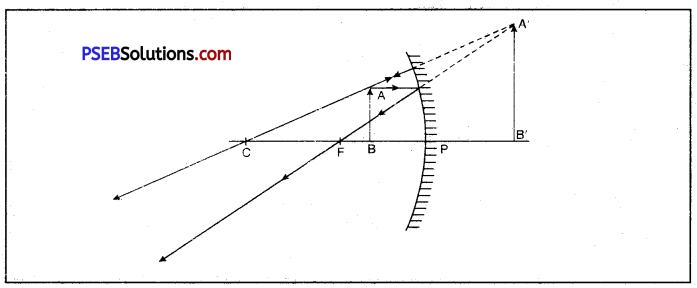This image is erect, enlarged. virtual and behind the mirror.

Question 8.
Name the type of mirror used in the following situations :
(b) Side/rear-view mirror of a vehicle.
(c) Solar furnace.
(a) Concave mirrors are used for head-lights of car to give concentrated parallel beam of light.
(b) Side/rear-view mirror of a vehicle is mostly convex mirror because it forms an erect, virtual and diminished image and therefore, gives a wider field of view of the traffic behind.
However, for judging the distance and speed of the rear vehicle, a plane mirror fixed inside the car.
(c) Solar furnaces use concave mirror. It is because parallel sun rays (coming from infinity) after reflection from concave mirror are converged at focus to produce much heat.

Question 9.
One half of a convex lens is covered with a black paper. Will this lens produce a complete image of the object? Explain your observation.
If lower half of a convex lens is covered with black paper no doubt it will form complete image but intensity (brightness) of image with half lens will be less as that with complete lens exposed. The nature, size and location of the image will be the same since light from all parts of the object reaches the exposed part of the lens.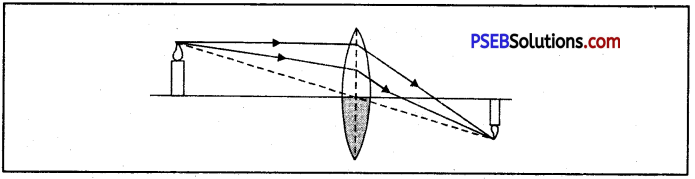Question 10.
An object 5 cm in length is held 25 cm away from a converging lens of focal length 10 cm. Draw the ray diagram and find the position, size and the nature of image formed.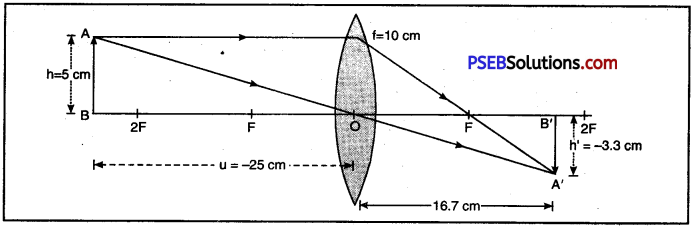Here, Length of the object (h) = 5 cm,
Image distance, v =?,
Object distance form the lens (u) = – 25 cm [Object distance is always negative]
h’ = ?; f = + 10cm [For convex lens, f is positive]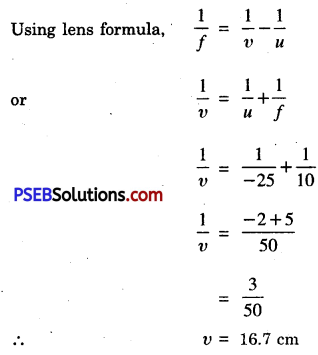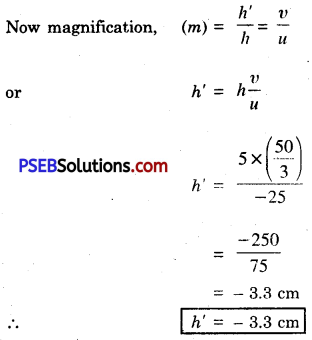Negative sign shows that the image is inverted, real and diminished (3.3 cm) which is formed at 16.7 cm distance on the right side of lens.

Question 11.
A concave lens of focal length 15 cm forms an image 10 cm from the lens. How far is the object placed from the lens? Draw ray diagram.
Distance of the image from the lens (V) = – 10 cm [Virtual image]
Focal length of concave lens (f) = – 15 cm [Focal length of concave lens is taken negative];
Distance of the object from the lens (u) = ?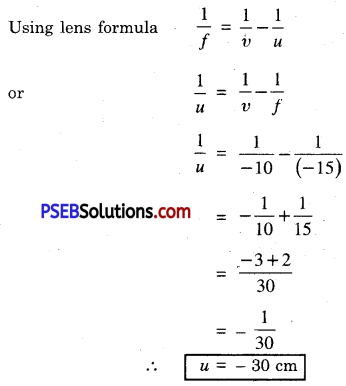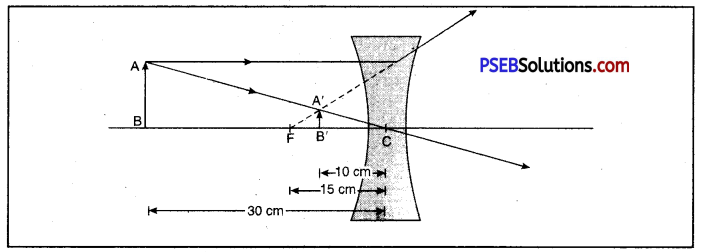Negative sign shows that the object is placed to the left side of lens.

Question 12.
An object is placed at a distance of 10 cm from a convex mirror of focal length of 15 cm. Find the position and nature of the image.
Distance of the object from the lens (u) = – 10 cm [u is always negative]
Focal length of the lens (f) = + 15 cm [convex mirror]
Distance of the image from the lens. (v) = ?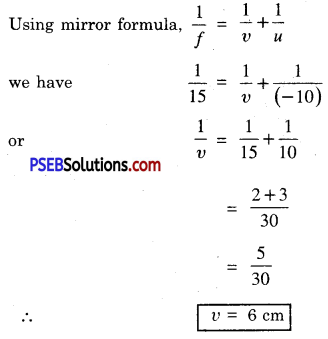Negative sign shows that the image is virtual and erect and is formed towards left of the mirror.

Question 13.
The magnification produced by a plane mirror is + 1. What does this mean?
It means that image produced by plane mirror is virtual, erect and of the same size as that of the object.Question 14.
An object 5.0 cm of length is placed at a distance of 20 cm in front of a convex mirror of radius of curvature 30 cm. Find the position of the image, its nature and size.
Here height of the object, h = 5.0 cm ;
u = – 20 cm [u is always negative]
Radius of curvature (r) = + 30 cm [convex mirror]
But f = $$\frac{r}{2}$$
= $$\frac{30}{2}$$
= + 15 cm

Height of the image h’ = ?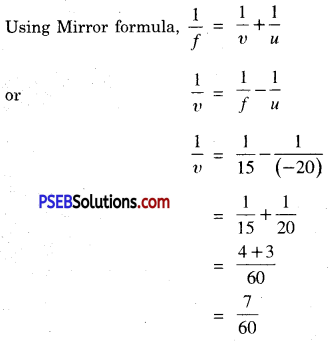Image distance from the mirror v = $$\frac{60}{7}$$ = 8.56 cm
Thus, the image is formed behind the mirror or to the right of the mirror.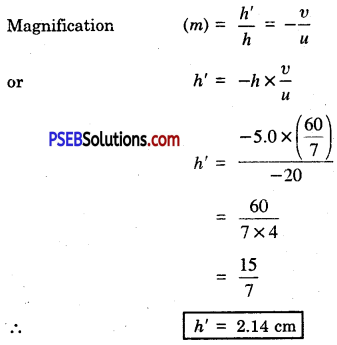Positive sign (+) indicates that the image is erect and formed above the principal axis.

Question 15 .
An object of size 7.0 cm is placed at 27 cm in front of a concave mirror of focal length 18 cm. At what distance from the mirror should the screen be placed, so that a sharp focussed image can be obtained? Find the size and the nature of the image.
Here h = 7.0 cm ; u = – 27 cm [u is always negative]
f = – 18 cm [concave mirror]
v =?; h’ =?

Using mirror formula ;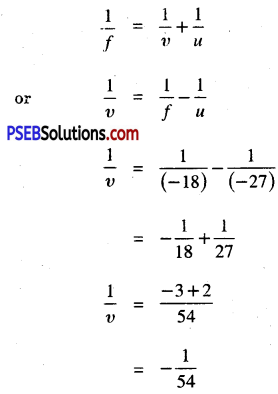∴ Image distance from the mirror v = – 54 cm
i. e., at 54 cm on the same side of the mirror as that of the object.
Now, Magnification (m) = $$\frac{h^{\prime}}{h}=-\frac{v}{u}$$
$$\frac{h^{\prime}}{7.0}=-\frac{(-54)}{(-27)}$$
= -2
h’ = – 2 × 7
∴ h’ = – 14 cm
Image is of size 14 cm i.e. magnified. Negative sign (-) shows that the image is real and inverted.

Question 16.
Find the focal length of a lens of power – 2.0 D. What type of lens is this?
Here, Power of lens (P) = – 2.0 D
Focal length of lens (f) =?
We have f = $$\frac{1}{P}$$
= $$\frac{1}{-2}$$
= – 0.5 m
∴ f = – 0.5 m = – 50 cm
Negative sign indicates that the lens is concave. Therefore, it is a divergent lens.

Question 17.
A doctor has prescribed a corrective lens of power + 1.5 D. Find the focal length of lens. Is prescribed lens diverging or converging?
Here power of lens (P) = + 1.5 D
Focal length of lens (f) =?
We know f = $$\frac{1}{P}$$
= $$\frac{1}{1.5}$$
∴ f = 0.67 m = 67 cm
The positive sign (+) shows that the lens is a convex lens which is diverging in nature.

Science Guide for Class 10 PSEB Light Reflection and Refraction InText Questions and Answers

Question 1.
Define the principal focus of concave mirror.
Principal focus: It is a point on the principal axis of a concave mirror where the rays parallel to principal axis meet after reflection from the mirror.

Question 2.
The radius of curvature of a spherical mirror is 20 cm. What is its focal length?
Spherical mirror may be either convex mirror or a concave mirror.

For convex mirror,
Radius of curvature (R) = + 20 cm
Focal length (f) =?

We know, f = $$\frac{R}{2}$$
f = $$\frac{20}{2}$$ = 10 cm

For concave mirrior,
Radius of curvature (R) = – 20 cm
Focal Length (f) = ?

We know, f = $$\frac{R}{2}$$
∴ f = $$\frac{-20}{2}$$ = -10 cm

Question 3.
Name a mirror which can give an erect and enlarged image of an object.
Concave mirror produces an erect and enlarged image when the object is placed between its pole and principal focus.

Question 4.
Why do we prefer a convex mirror as back view mirror in vehicles?
Preference of convex mirror as rear view mirror in vehicles. We prefer to have convex mirror as a rear view mirror in vehicles because of the following reasons :

• It produces an erect image of the object (traffic behind).
• The size of the image formed is much smaller than the size of the object.
• It has a wider field of view.

Question 5.
Find the focal length of a convex mirror whose radius of curvature is 32 cm.
Radius of curvature (R) = + 32 cm [for convex mirror, R is +ve]
Focal length (f) =?

We know, f = $$\frac{R}{2}$$

∴ f = $$\frac{32}{2}$$ = 16 cm

Question 6.
A concave mirror produces three times magnified (enlarged) real image of an object placed at 10 cm in front of it. Where is the image located?
Magnification (m) = – 3 [for real image m is taken as negative]
u = – 10 cm v =? ; [u is always negative]

We know, m = $$-\frac{v}{u}$$
-3 = $$-\frac{v}{(-10)}$$
∴ v = – 30 cm
Since, the value of v is negative, therefore, the image is formed at 30 cm on the same side (to the left) of the mirror as that of the object.

Question 7.
A ray of light travelling in air enters obliquely into water. Does the light ray bend towards normal or away from normal? Why?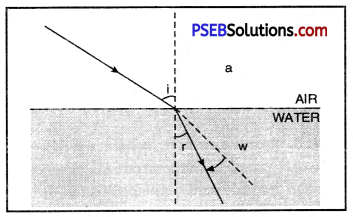anw = $$\frac{\sin i}{\sin r}$$
Since anw > 1,
sin i > sin r
or i > r
or r < i
Hence, a ray of light travelling from optically rarer medium (air) to an optically denser medium (water), bends towards normal.

Question 8.
Light enters from air to glass having refractive index 1.50. What is speed of light in glass? Speed of light in vacuum is 3 × 108 m s-1.
Refractive index of glass (µ) = 1.50
Speed of light in vacuum (c) = 3 × 108 m s-1

We know, Refractive index = $$\frac{\text { Speed of light in vacuum }(c)}{\text { Speed of light in glass }\left(v_{g}\right)}$$
1.50 = $$\frac{3 \times 10^{8}}{v_{g}}$$
or vg = $$\frac{3 \times 10^{8}}{1.50}$$
vg = 2 × 108 m s-1

Question 9.
Find out from table 10.3 of the text-book, the medium having highest optical density. Also find the medium with lowest optical density.

• Optical density of a medium depends upon its refractive index. The higher the refractive index, higher the optical density and vice versa.
• From the data available in the table it is apparent that diamond is having highest refractive index (µ = 2.42) and air is having lowest refractive index (µ = 1,0003).Question 10.
Refractive indices of kerosene, turpentine and water are 1.44,1.47 and 1.33 respectively. In which material does the light travel fastest and why?
Refractive indices of kerosene, turpentine and water are 1.44, 1.47 and 1.33 respectively. We know that velocity of light in a medium is inversely proportional to refractive index of the medium.

Therefore, light will travel fastest in water (having least refractive index i.e. µ = 1.33) and slowest in turpentine oil (having maximum refractive index i.e., µ = 1.47.)

Question 11.
The refractive index of diamond is 2.42. What is the meaning of this statement?
We know, Refractive index of diamond = $$\frac{\text { Velocity of light in vacuum }}{\text { Refractive index of diamond }}$$
or
Velocity of light m diamond = $$\frac{\text { Velocity of light in vacuum }}{\text { Refractive index of diamond }}$$
The statement means that velocity of light in diamond is $$\frac{1}{2.42}$$ times the velocity of light in vacuum.
i.e., vD = $$\frac{3 \times 10^{8}}{2.42}$$ m s-1
= 1.24 × 108 m s-1-1
In other words, light travels 2.42 times faster in vacuum than in diamond.

Question 12.
Define 1 dioptre of power of a lens.
Or
What is power of Lens? Give the commercial unit of power.

• Power of Lens: It is defined as the ability of a lens to bend incident light rays.
• It is measured by taking reciprocal of focal length measured in metres.
• The power of a lens is said to be 1 dioptre if its focal length is one metre.

Question 13.
A convex lens forms a real and inverted image of a needle at a distance of 50 cm from it. Where is the needle be placed in front of the convex lens if the image is equal to size of the object? Also, find the power of the lens.
Here u =?, f =?, P =?
v = + 50 cm [for real image u is taken as positive]
m = -1 [Real image is always inverted]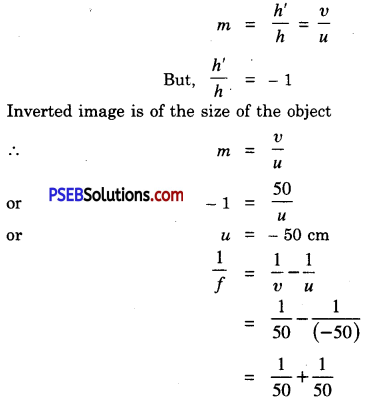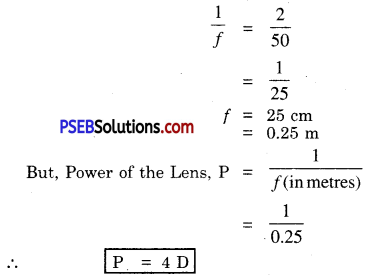Question 14.
Find the power of a concave lens of focal length 2 metres.
Power of lens P = $$\frac{1}{f}$$
= $$\frac{1}{(-2)}$$